Back to index
Multiple linear regression for the HP-42S

By Namir Shammas

MLRAN is a program that performs multiple linear regression and provides the following results:
1. Coefficients of multiple linear regressions
2. Coefficient of determination and its adjusted value
3. The regression ANOVA table
The program uses matrix operations to calculate the regression coefficients column matrix:

C = inv(X' X) * (X' Y)

Where X' is the transpose of matrix X. The program calculates the ANOVA table entries:

SSR = C' X' Y - sum(Y)^2 / N
SSE = Y' Y - C'X'Y
SST = Y' Y - sum(Y)^2 / N
DF Residuals = K
DF Error = N - K - 1
DF Total = N - 1
MSR = SSR / K
MSE = SSE / (N - K - 1)
Fstat = MSR / MSE
R2 = SSR / SST
R2adj = 1 - (1 - R2) (N - 1) / (N - K - 1)

Where N is the number of observations and K is the number of independent variables.

Usage:

1. Before you start the program you must enter the dependent variable's observations is a column matrix (call it MY) and the independent variables' observations in a matrix (call it MX). The first column of this matrix must be full of ones. The second column in the matrix contains the data for the first independent variable. The third column in the matrix contains the data for the second independent variable, and so on. You can create and edit these matrices in the stack with or without storing them. I recommend storing them to be on the safe side.
2. Push matrix MY into the stack (if no in the stack already).
3. Push matrix MX into the stack (if no in the stack already)
4. Start the program by executing the command XEQ "MLRAN"
5. The program prints the regression results.
6. To view or review the output on the display execute XEQ "VWRS". The program places the matrix MC on the stack and executes an EDIT command. The program halts while you are inspecting the matrix elements. Once you are done, press R/S to view the other results. Press R/S to view the next results. The program beeps when you there are no more results to display.
7. To clear the program's variables except variables MX, MY, and MC, execute the command XEQ "CLRANV". Please remember that once you execute this step you cannot execute step 6 since the variables for the ANOVA table are deleted.
Note: The program stores various data in the following variables:

MY      Dependent variable's observations.
MX      Independent variable's observations.
MC      Regression coefficients.
R2      Coefficient of determination.
SSR     Sum of squares to due regression
SSE     Sum of squares due to error
SST     Total sum of squares of dependent variable.
DFR     Degrees of freedom for regression
DFE     Degrees of freedom of error
DFT     Total degrees of freedom
MSR     Mean sum of residuals
MSE     Mean sum of errors
FSTAT   F statistics

Example:

Given the following matrix of observations:

X     Y    Z    T
2     2    34   101.010
3     7    26   81.191
4     3    76   14.439
5     5    43   61.667
6     9    23   108.915
7     2    15   113.257

Fit the model:

T = c0 + c1 X + c2 Y + c3 Z

The column matrix MY is:

101.010
81.191
14.439
61.667
108.915
113.257

The matrix MX is:

1 2 2 34
1 3 7 26
1 4 3 76
1 5 5 43
1 6 9 23
1 7 2 15

Enter these matrices either in the stack (MY in register y and MX in register X) and then execute the program "MLR".

XEQ "MLRAN"
MC=       [ 4x1 Matrix ]
1:1=            152.0517
2:1=             -0.9443
3:1=             -1.2403
4:1=             -1.7125
R2=               0.9182
ANOVA TABLE
SSR=          6,445.0129
SSE=            574.2363
SST=          7,019.2492
DFR=              3.0000
DFE=              2.0000
DFT=              5.0000
MSR=          2,148.3376
MSE=            287.1182
FSTAT=            7.4824

The fitted model is:

T = 152.0517 -0.9443 X -1.2403 Y -1.7125 Z

The coefficient of determination for the above model and data is 0.9182.

To review the results on the LCD, execute command XEQ "VWRS". The output starts with an EDIT command that allows you to inspect the column matrix for the regression coefficients. Once you are done with the inspection, press R/S to view the other results as shown below:

XEQ "VWRS"
1:1=152.0517              -> (view next element)
2:1=-0.9443               -> (view next element)
3:1=-1.2403               -> (view next element)
4:1=-1.7125
EXIT

RUN
R2=0.9182
RUN
RUN
SSR=6,445.0129
RUN
SSE=574.2363
RUN
SST=7,019.2492
RUN
DFR=3.0000
RUN
DFE=2.0000
RUN
DFT=5.0000
RUN
MSR=2,148.3376
RUN
MSE=287.1182
RUN
FSTAT=7.4824
RUN
(audible beep)

Technical Notes:

Memory Usage:

MY      Dependent variable's observations.
MX      Independent variable's observations.
MC      Regression coefficients.
R2      Coefficient of determination.
SSR     Sum of squares to due regression
SSE     Sum of squares due to error
SST     Total sum of squares of dependent variable.
DFR     Degrees of freedom for regression
DFE     Degrees of freedom of error
DFT     Total degrees of freedom
MSR     Mean sum of residuals
MSE     Mean sum of errors
FSTAT   F statistics
N       Number of observations
K       Number of independent variables
MM      used
SY      used

Label Usage:

MLRAN  Main program
CLRANV Clear ANOVA and miscellaneous variables
VWRS   View results
00     Used

Binary files for emulators:
Raw binary: mlran.raw  Binary for HP-42X: mlran.42x (HP-48) mlran49.42x (HP-49)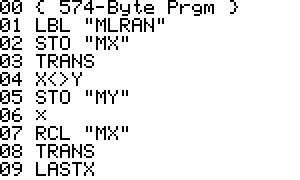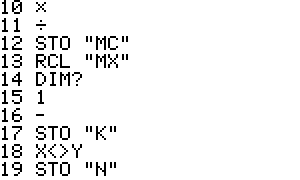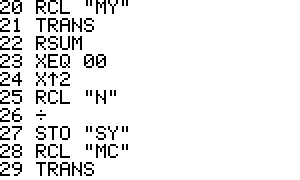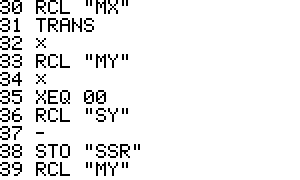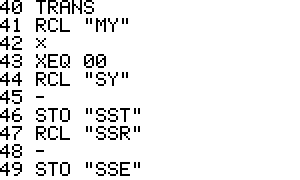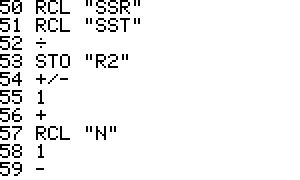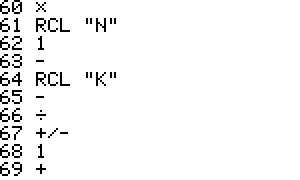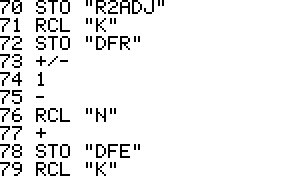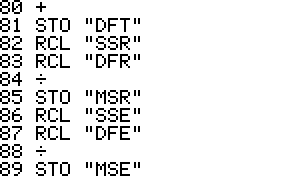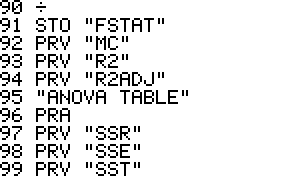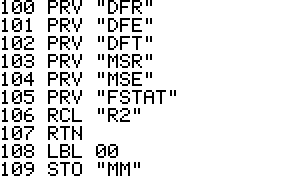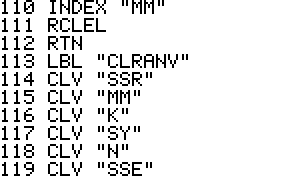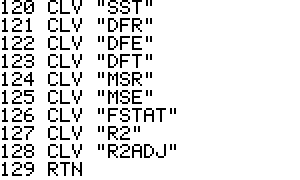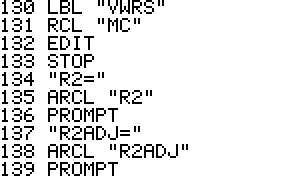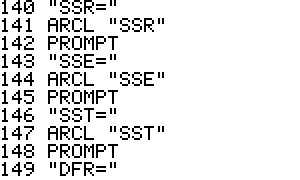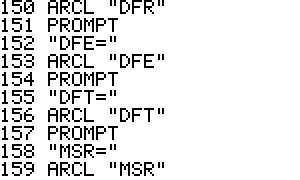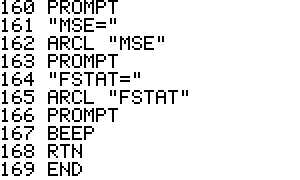Generated by 42s2html - 15 June 2005, 11:38